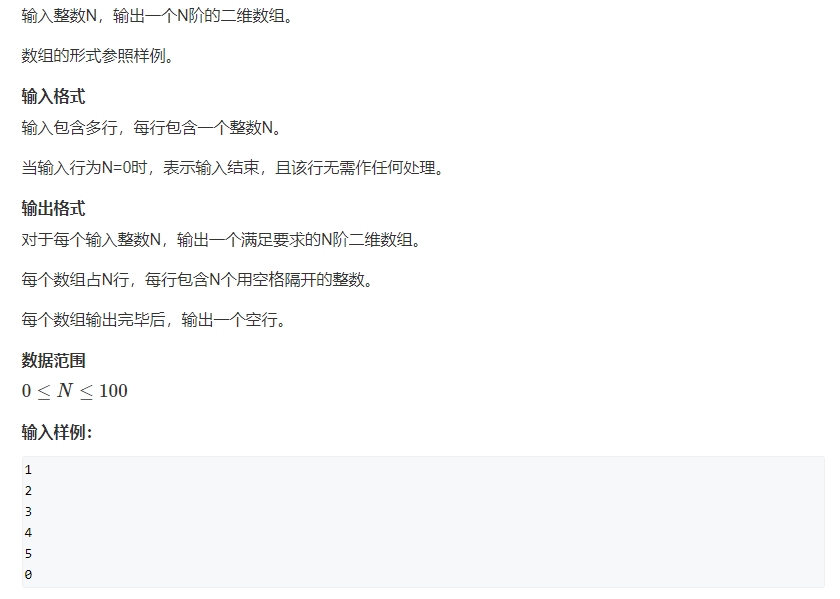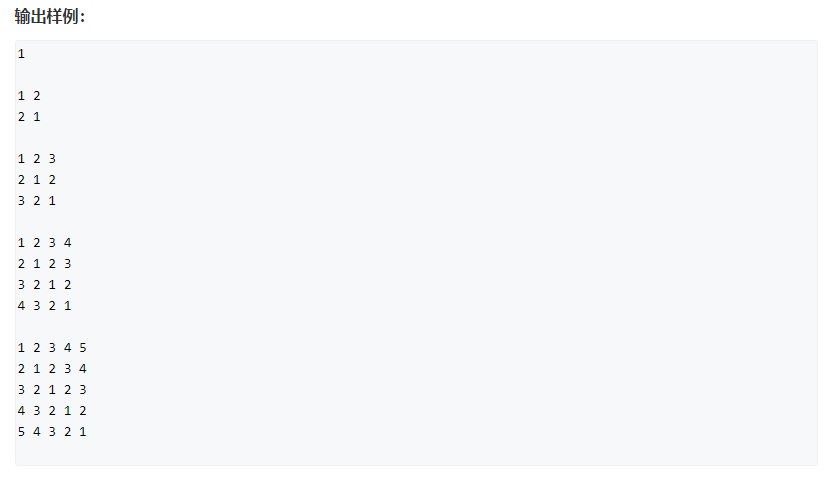# 前言# 题目

• 输入包含多行，每行包含一个整数N。
• 当输入行为N=0时，表示输入结束，且该行无需作任何处理。

• 对于每个输入整数N，输出一个满足要求的N阶二维数组。
• 每个数组占N行，每行包含N个用空格隔开的整数。
• 每个数组输出完毕后，输出一个空行。

• 0 ≤ N ≤ 100 0≤N≤100
输入样例：
1
2
3
4
5
0

1

1 2
2 1

1 2 3
2 1 2
3 2 1

1 2 3 4
2 1 2 3
3 2 1 2
4 3 2 1

1 2 3 4 5
2 1 2 3 4
3 2 1 2 3
4 3 2 1 2
5 4 3 2 1# 详细题解

#### 写法1 O ( n 2 ) O(n^2)

#include <iostream>
#include <cstring>
#include <algorithm>

using namespace std;

const int N = 105;

int n;

int main()
{
while (cin >> n, n)
{
for (int i = 1; i <= n; ++ i )
{
for (int j = i; j >= 1; -- j ) cout << j << " ";
for (int j = i + 1; j <= n; ++ j ) cout << j - i + 1 << " ";
cout << endl;
}
cout << endl;
}

return 0;
}#### 推导证明#### 写法2 O ( n 2 ) O(n^2)

#include <iostream>
#include <cstring>
#include <algorithm>

using namespace std;

const int N = 105;

int n;
int a[N][N];

int main()
{
while (cin >> n, n)
{
for (int i = 1; i <= n; i ++ )
for (int j = i, k = 1; j <= n; j ++, k ++ )
{
a[i][j] = k;
a[j][i] = k;
}

for (int i = 1; i <= n; i ++ )
{
for (int j = 1; j <= n; j ++ )
cout << a[i][j] << ' ';
cout << endl;
}
cout << endl;
}

return 0;
}#### 推导证明#### 写法3 O ( n 2 ) O(n^2)

#include <iostream>
#include <cstring>
#include <algorithm>

using namespace std;

const int N = 105;

int n;
int main()
{
while (cin >> n, n)
{
for (int i = 1; i <= n; i ++ )
{
for (int j = 1; j <= n; j ++ )
cout << abs(i - j) + 1 << ' ';
cout << endl;
}
cout << endl;
}

return 0;
}#### 推导证明# 总结02-07170703-0930万+
02-132790
01-29800
10-062312
02-03614
01-30743
01-271541
02-09540
01-14378
02-06557
02-012307
02-0886
02-252342
05-264496
02-27289
07-04109
04-051万+
12-31
©️2020 CSDN 皮肤主题: 酷酷鲨 设计师:CSDN官方博客